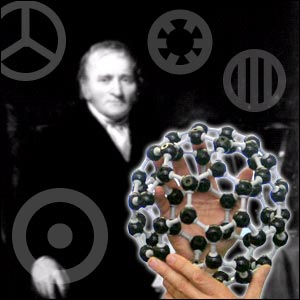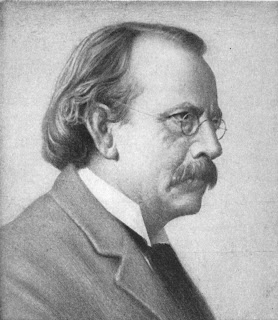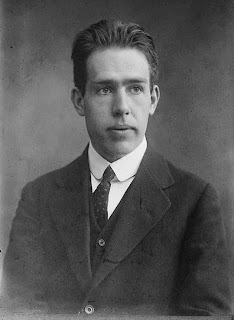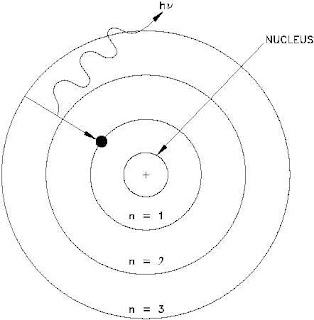## Thursday, 16 February 2012

### HISTORY OF CONCEPT OF THE ATOM AND ITS STRUCTURE:

HISTORY OF CONCEPT OF THE ATOM AND ITS STRUCTURE:

The word "Atom"  has   came from the Greek word "atomos" .Atomos  means indivisible . Scientist Dalton originated this concept but it is obselete after invention of electron, proton and neutron particles. It is observe that when any metal is heated it emits electrons.Dalton-Atomic-Model

Gradually after many scientific observation  it is proved that every matter have electrons in their atom. Usually an atom is non-ionic therefore it have equal amount of negative and positive ion .Then the main question arises among the scientist that how these ions are placed inside the atom. First model to define that how ions are placed inside the atom is Thomson`s Plum pudding model.J.J.Thomson
THOMSON`S PLUM PUDDING MODEL:
- 8
According to him an Atom is 10      cm  diameter positively charged sphere where positive charge is equally distributed. The electrons are embedded within this sphere like raisin within the pudding, among the positively charged cloud of sphere.Plum-Pudding-Model J.J.Thomsom
RUTHERFORD MODEL:Ernest-Rutherford

Experiment:

In 1911 Rutherford obserb the  deflection of alpha(a) particles on metal plate. Alpha particles is similar to positively charged helium atom. Alpha particles are emitted from the nucleus of radioactive subtance.Alpha particles are very tiny but its mass is greater than the electrons. When Rutherford hit a metal plate with Alpha particles ,he observed that most of the alpha particles pass through the metal plate without cause any harm on the plate.

1) Most of the alpha particles deflection is very minimum.

2) Few alpha particles is actually deflected.

3) Very few among the few alpha particles deflected more than 90 degree.

4) Very tiny amount of alpha particles deflected back at 180 degree from the metal Plate.

Rutherford`s deduction:

Rutherford cannot explain his observation by previously known Thomson`s model. Therefore he proposed another new model of atom to explain his observation.According to his new model he propose that most of the positive charge or mass of a matter present in a very small central area which is call the nucleus.The electrons are present around the central nucleus.
1) The most of the positively charged alpha particles  penetrate the atom on its surrounding area other than the nucleus and they feel very low repelling force therefore they have minimum deflection.

2) When the alpha particles goes through nearer part  of the nucleus they feel much higher repelling force and deflected highly.

3) When the alpha particle go directly to the nucleus, they feel huge repulsive force and reflected back to their inital position.

-12
It is proven after calculation that in the third case the alpha particles must be within 10      cm nearer to the central nucleus
-12
therefore diameter of the central nucleus must not higher than the 10       cm. Again it is known according the gas law that
- 8
the diameter of nucleus is 10     cm . So the most of the part of the atom is empty.

According to Rutherford model electrons are within the atom placed in circular area around the nucleus. The electrons are not static because negatively charged electrons are attracted toward positively charged nucleus  which cannot be possible (if the electrons are goes towards the nucleus it will fuse together and all matter will be destroyed). Therefore it is assume that all the electrons are move in a circular orbit around the central nucleus and their attraction force is provide its centripetal force.This model is similar to the Sun, Planets and orbits (Sun is like  a nucleus in atom and planets are similar like electrons which are circulate around the nucleus or sun in their orbit). Therefore it is also known as Rutherford planetary atom model.Planetary-Atom-Model-Rutherford

Defects of Rutherford`s Model:

According to the electromagnetic law ionized moving paricles must release its energy in form of electromagnetic radiation.Therefore moving electrons around the nucleus must continuously release its energy. The radius and speed of electron`s circular orbit are gradually decrease . Therefore the electrons are gradually come nearer to the atom`s nucleus.It
- 8
is calculated that an electron release all its energy within 10     seconds and fall on its nucleus. This defects of unstable atom is corrected by the Rutherford`s student Niels Bohr in the year 1913.

NIELS BOHR ATOMIC MODEL:Niels Bohr
In his model, Niels Bohr applied Plank and Einstein`s quantum law instead of electromagnetic and mechanics laws. His model is build on the three basic foundation , which are given below:-

1) Electrons cannot circulate around the nucleus on any orbit.They must circulate  along a fixed orbit, which paths are defined by specific quantum law. The specific orbit which have fully multiplied by electron`s angular momentum(mass* Velocity) = h/2p (Here h = Plank`s constant) are known  permissible orbit . These permissible orbit are known as stable or stationary or quantum orbit.Model- Of-Niel Bohr
A) Calculation of theory of model:

Let assume that an electron of mass m is circulating around the orbit having r radius and the angular velocity of the electron is w.

Then the momentum of electron J = Iw
2                                                         v
Here I = mr     is electron`s inertia and w = ------
r

2         v
Therefore  J = mr     * -------  = m v r
r
nh
J = m v r = --------
2p

Here n is full number like 1,2,3 etc.They are known as principal quantum number. Different orbit has different principal  quantum number(n). The electron`s energy increases with the increasing amount of principal quantum number (n).

2) The electrons not release its energy until it orbiting around the  quantum orbit.

3) When electrons of an atom are jump form higher energy orbit to lower energy orbit , atom release enegy. When electron jump from lower energy to higher energy , atom sucks energy.

During energy radiation or suction the energy of electron on initial orbit and last orbit are E1 and E2 respectively then the energy of photon during radiation and suction is:-

hv = E1~ E2 ( here v = frequency of radiation,  h = Planck`s constant)

QUANTUM NUMBERS:

The discoveries of wave Particle duality by Broglie and the uncertainty principle by Heisenberg have completely changed the thinking about the behaviour of electrons in an atom .The behaviour of electrons in an atom is described by quantum theory .Each electron in an atom is characterized by a mathematical equation (Known as wave function) Whose square value at a given point gives the probability of finding the electron at that point .These wave functions are obtained by solving the Appropiate Schrodinger equation.Each wave function constitutes an orbital in an atom and depends on three quantum numbers n,l and m as describe in the following:

Principal Quantum Number: (Symbol: n)

Each principal quantum number represents a major energy shell.It can have integral values 1, 2, 3, ....  .The energy shells are represented by the symbols K, L, M, ... depending upon the values of n equal to 1, 2, 3,..... , respectively. For one electron system, the expression of energy is the same as that obtained in Bohr`s theory.For a multi electron system, the energy of an orbital depends on the two quantum numbers n and l.

Azimuthal quantum Number:(Symbol: l)

Each Azimuthal quantum number represents a sunshell within the major energy shell .The values of l are 0, 1, 2, ...,(n-1) and these are designated by the symbols s, p, d, f, g, ...., respectively .This quantum number also represents the quantized values of angular momentum of the electron in an orbital through the expression:

h
L =V l(l+1)     *  ---------
2p

This quantum number also describes the shape of an orbital which, according to the quantum theory , is the space around the nucleus where there exits 90 to 95% probability of finding the electron .The orbital with l = 0 is spherical , for
l = 1, the shape is dumb-bell and for l = 2, the shape is double dumb-bell.Atom-Otto-Hahn.
Spin Quantum Number:Symbol (ms):

The electron acn also spin around its own axis . Two types of spinning, namely clockwise and anticlockwise is
_____
possible.This spinning produces angular momentum equal to V s(s+1) (h/2p), where s = 1/2 the direction of angular momentum is governed by the quantize value if z component of angular momentum which is given as ms (h/2p) where
ms is either equal to + 1/2 (clockwise spinning) or -1/2 (anti clockwise spinning).Electron Orbital Spin